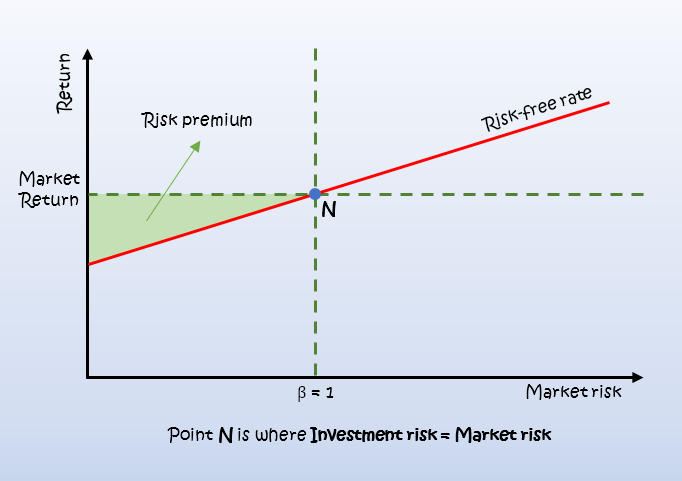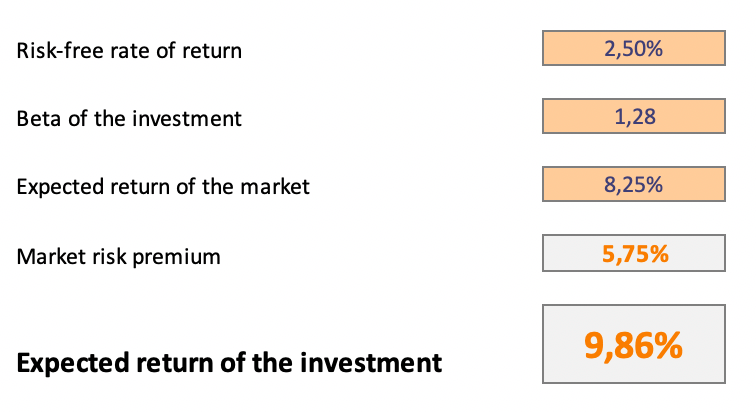# Introduction

The Capital Asset Pricing Model (CAPM) shows us the relationship between systematic risk for an investment and the expected return on it.

Analysts and financial professionals use the model widely for pricing risky investments and generating expected returns for assets, considering the risk and cost of capital.

The CAPM goal is to see if an investment is valued fair by comparing risk and time value to the expected return.

# Capital Asset Pricing Model (CAPM)

To calculate the CAPM we use the following formula:Where:

• Ri is the expected return on the investment;
• Rf is the risk-free rate;
• βi is the beta of the investment; and
• Rm is the expected return of the market.

Investors want compensation for the time value of money and the additional risk they take for a riskier investment. The risk-free rate covers the time-value of money. The other components of the CAPM equation are for the additional risk taken by the investors.

## Risk-free rate (Rf)

The risk-free rate is usually the return rate on government bonds. It is common to use 10-year bonds because they’re most heavily quoted and most liquid. It is also a good idea to pick bonds that have a similar maturity to the investment we are reviewing.

## Beta (βi)

The beta is a measure of how much risk the investment will add to our portfolio, a measure of its returns’ volatility. It shows the fluctuations of the price changes relative to the overall market.

If the beta is above 1.0, it means the investment is riskier than the market average. On the other hand, if the beta is below 1.0, the investment is less risky than the market average and will decrease the overall risk of our portfolio. Also, when the beta is 1.0, the expected return is equal to the average market return.

Most investors prefer to use betas already calculated by third parties like Yahoo Finance and others. We need to be aware that beta can be different based on the period it’s calculated over.

We can also calculate the beta ourselves, as a straight-line statistical regression model on data points illustrating price changes of a risky asset versus those of a market index.

## Market Risk Premium (Rm – Rf)

We multiply the beta by the market risk premium. The market risk premium represents the expected return from the market, above the risk-free rate.

The more volatile a market or an investment class is, the higher the market risk premium will be.

## Expected return (Ri)

When we add all the components of the equation, we get the desired yield of the investment or the discount rate we can use to find the present value of its future cash flows.

## Graphical representation

The main underlying concept of the Capital Asset Pricing Model is that the expected return is equal to the risk-free return, increased by a risk premium. To visualize this, we can graphically present the model.# Using the Capital Asset Pricing Model

We use the model widely in the finance industry. It is critical in calculating the weighted average cost of capital (WACC), as CAPM computes the cost of equity. WACC is used extensively in financial modeling, as it can be used to find the net present value of future cash flows. From there, we can calculate the enterprise value and finally, the fair value of the business/investment.

# Example CAPM calculation

Let’s illustrate the model by example.

We are currently looking at an investment opportunity, that will cost us 35 thousand euro. It will bring us a 10% annual dividend for the next ten years, after which we can release the investment by selling it for 30 thousand euros. The risk-free rate of return derived from 10-year government bonds is 2,50%. The beta of the investment is 1,28, which means it’s riskier relative to the market and will increase our portfolio risk. The expected return on investments for the market is 8,25%. Using this information, we can calculate the expected rate of return on the investment.Now that we have the expected rate of return, we can use it to discount the expected future cash flows and derive the present value of the future cash flows of the investment.Using the information above we calculate the expected cash flows for the next ten years, including the release value at the sale on the tenth year. We then discount those using the above calculated expected return rate.Calculating the total of the present values of the expected future cash flows and comparing them to the investment price, we can see that it is overpriced and is therefore not an attractive investment opportunity.# Conclusion

The CAPM (Capital Asset Pricing Model) determines if an investment is reasonably priced. It is flawed as far as it relies on risk and returns distributions, the behavior of other investors, and some fundamentals of the market, that do not exist in the same form in reality. However, the concepts behind CAPM can help us understand the connection between expected returns and risk and thus assist us in improving our decision-making process when deciding whether to add an investment to our portfolio.## FCCA, FMVA

Hi! I am a finance professional with 10+ years of experience in audit, controlling, reporting, financial analysis and modeling. I am excited to delve deep into specifics of various industries, where I can identify the best solutions for clients I work with.

In my spare time, I am into skiing, hiking and running. I am also active on Instagram and YouTube, where I try different ways to express my creative side.

The information and views set out in this publication are those of the author(s) and do not necessarily reflect the official opinion of Magnimetrics. Neither Magnimetrics nor any person acting on their behalf may be held responsible for the use which may be made of the information contained herein. The information in this article is for educational purposes only and should not be treated as professional advice. Magnimetrics and the author of this publication accept no responsibility for any damages or losses sustained in the result of using the information presented in the publication.